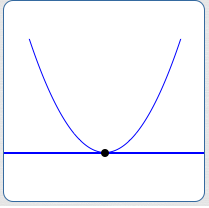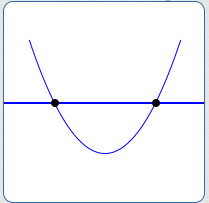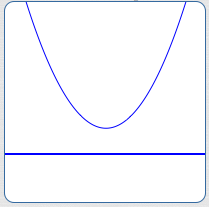﻿ The Quadratic Formula Revisited; the Discriminant

# THE QUADRATIC FORMULA REVISITED; THE DISCRIMINANT

• PRACTICE (online exercises and printable worksheets)

The quadratic formula and discriminant were introduced in a prior section in the Algebra II curriculum.

Quickly read through this earlier section and do plenty of the online exercises to make sure that you understand the concepts there.

For your convenience, this current section gives an in-a-nutshell review of the quadratic formula, discriminant, and related concepts.

 REVIEW the quadratic formula, the discriminant, related concepts QUADRATIC EQUATIONS: A quadratic equation is an equation that can be written in the form $\,ax^2 + bx + c = 0\,$, where $\,a\,$, $\,b\,$ and $\,c\,$ are real numbers, with $\,a\ne 0\,$. Thus, a quadratic equation must have an $\,x^2\,$ term; it is allowed to have an $\,x\,$ term and a constant term. STANDARD FORM OF A QUADRATIC EQUATION: The form ‘$\,ax^2 + bx + c = 0\,$’ is called the standard form of the quadratic equation. Thus, in standard form, a zero appears on the right-hand side; on the left, the $\,x^2\,$ term comes first, followed by the (optional) $\,x\,$ term and the (optional) constant term. STANDARD FORM IS NOT UNIQUE: Standard form is not unique. For example, ‘$\,3x^2 - 2x + 1 = 0\,$’ and ‘$\,-3x^2 + 2x - 1 = 0\,$’ are equivalent equations; both are in standard form. If uniqueness is desired, the following additional requirements can be imposed: the greatest common factor of all nonzero coefficients is $\,1\,$ $a > 0$ THE QUADRATIC FORMULA: Let $\,a\,$, $\,b\,$ and $\,c\,$ be real numbers, with $\,a\ne 0\,$. Then, the following two equations are equivalent—they are true at the same time, and false at the same time: $$\begin{gather} ax^2 + bx + c = 0\cr\cr \color{red}{x = \frac{-b\pm\sqrt{b^2 - 4ac}}{2a}}\cr \color{red}{\text{THE QUADRATIC FORMULA}} \end{gather}$$ The quadratic formula is derived here. The plus-or-minus sign ($\,\pm\,$) that appears in the quadratic formula provides a convenient shorthand. Otherwise, the quadratic formula would look like this: $$\color{red}{x = \frac{-b +\sqrt{b^2 - 4ac}}{2a} \qquad \text{ or } \qquad x = \frac{-b -\sqrt{b^2 - 4ac}}{2a}}$$ The quadratic formula gives all complex solutions to the quadratic equation $\,ax^2 + bx + c = 0\,$. THE DISCRIMINANT OF A QUADRATIC EQUATION: See that expression ‘$\,b^2 - 4ac\,$’ under the square root in the quadratic formula? It is called the discriminant. As shown below, it helps us to discriminate between the various types of solutions to a quadratic equation. The graph of every quadratic function $\,y = ax^2 + bx + c\,$,   $\,a\ne 0\,$, is a parabola. The parabola has different $x$-intercepts based on different values of the discriminant: $b^2 - 4ac = 0$ exactly one $x$-intercept$b^2 - 4ac > 0$ two different $x$-intercepts$b^2 - 4ac < 0$ no $x$-intercepts## NATURE OF SOLUTIONS TO A QUADRATIC EQUATION WITH REAL COEFFICIENTS

There are three types of solution sets possible for a quadratic equation $\,ax^2 + bx + c = 0\,$, $\,a\ne 0\,$.
These solution sets are determined by the discriminant, $\,b^2 - 4ac\,$: whether it is zero, positive, or negative.
The three situations are summarized in the table below.

 SOLUTIONS OF $ax^2 + bx + c = 0\,$,   coefficients are real numbers, $a\ne 0$ value of the discriminant $b^2 - 4ac = 0\$ $b^2 - 4ac > 0\$ $b^2 - 4ac < 0\$ nature of solution set exactly one real number solution two different real number solutions no real number solutions; a complex conjugate pair the solution(s) are: $$\displaystyle\,x = -\frac{b}{2a}$$ $\displaystyle\,x = \frac{-b + \sqrt{b^2 - 4ac}}{2a}$ or $\displaystyle\,x = \frac{-b - \sqrt{b^2 - 4ac}}{2a}$ \begin{align} x &= \frac{-b + i\sqrt{-(b^2 - 4ac)}}{2a}\cr &= -\frac{b}{2a} + i\frac{\sqrt{-(b^2-4ac)}}{2a} \end{align} or \begin{align} x &= \frac{-b - i\sqrt{-(b^2 - 4ac)}}{2a}\cr &= -\frac{b}{2a} - i\frac{\sqrt{-(b^2-4ac)}}{2a} \end{align} Note: Since $\,b^2 - 4ac < 0\,$, we have $\,-(b^2 - 4ac) > 0\,$. Therefore, $\,\frac{\sqrt{-(b^2-4ac)}}{2a} \ne 0\,$, so these two solutions have the same real part and opposite nonzero imaginary parts, hence form a complex conjugate pair.

The quadratic expression $\ ax^2 + bx + c\$ ($\,a \ne 0\,$) is called irreducible if $\ b^2 - 4ac < 0\$.

Equivalently, the quadratic expression $\ ax^2 + bx + c\$ ($\,a \ne 0\,$) is called irreducible if the equation $\,ax^2 + bx + c = 0\,$ has no real number solutions.

In this case, the trinomial $\,ax^2 + bx + c\,$ cannot be factored using real numbers.
It can, however, be factored using the complex conjugate pair that is given by the quadratic formula.
This idea is explored in a future section.

## EXAMPLES: Connection Between Zeroes and Factors

Recall the beautiful relationship between the zeroes of a polynomial and its factors:

$k\,$ is a zero             if and only if             $x-k\,$ is a factor

Indeed, the only factor that the zeroes don't give is a constant factor, which we often need to provide ourselves.
Some examples follow.

EXAMPLE:
Find a quadratic function with zeroes $\,2\,$ and $\,-3\,$;
the coefficient of the $\,x^2\,$ term should equal $\,5\,$.

SOLUTION:
Since $\,2\,$ is a zero, $\,x - 2\,$ is a factor.
Since $-3\,$ is a zero, $\,x - (-3) = x + 3\,$ is a factor.
Since the coefficient of the $\,x^2\,$ term must be $\,5\,$, we need a constant factor of $\,5\,$.
(Note that this constant factor does not change the zeroes.)

The function $\,f\,$ defined below is the desired quadratic function: $$\,f(x) = 5(x-2)(x+3) = \ldots = 5x^2 + 5x - 30\,$$

EXAMPLE:
Find a quadratic function with real coefficients that has exactly one real zero, $x = 4\,$.
The coefficient of the $\,x\,$ term should equal $\,7\,$.

SOLUTION:
Since $\,4\,$ is a zero, $\,x - 4\,$ is a factor.
To get a quadratic function with no different zero, we need two factors of $\,x - 4\,$.
Thus, every function of the form $\,k(x-4)(x-4) = \ldots = kx^2 - 8kx + 16k\,$ satisfies the zero requirement.
For the coefficient of the $\,x\,$ term to equal $\,7\,$, we need $\,-8k = 7\,$, so $k = -\frac{7}{8}\,$.

The function $\,f\,$ defined below is the desired quadratic function: $$\,f(x) = -\frac{7}{8}(x-4)(x-4) = \ldots = -\frac{7}{8}x^2 + 7x - 14\,$$

Master the ideas from this section